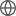Old Web
English
We report the discovery of the massive hot Jupiter NGTS-13b by the Next Generation Transit Survey (NGTS). The V = 12.7 host star is likely in the subgiant evolutionary phase with log g$_{*}$ = 4.04 $\pm$ 0.05, T$_{eff}$ = 5819 $\pm$ 73 K, M$_{*}$ = 1.30$^{+0.11}_{-0.18}$ M$_{\odot}$, and R$_{*}$ = 1.79 $\pm$ 0.06 R$_{\odot}$. NGTS detected a transiting planet with a period of P = 4.12 days around the star, which was later validated with the Transiting Exoplanet Survey Satellite (TESS; TIC 454069765). We confirm the planet using radial velocities from the CORALIE spectrograph. Using NGTS and TESS full-frame image photometry combined with CORALIE radial velocities we determine NGTS-13b to have a radius of R$_{P}$ = 1.142 $\pm$ 0.046 R$_{Jup}$, mass of M$_{P}$ = 4.84 $\pm$ 0.44 M$_{Jup}$ and eccentricity e = 0.086 $\pm$ 0.034. Some previous studies suggest that $\sim$4 M$_{Jup}$ may be a border between two separate formation scenarios (e.g., core accretion and disk instability) and that massive giant planets share similar formation mechanisms as lower-mass brown dwarfs. NGTS-13b is just above 4 M$_{Jup}$ making it an important addition to the statistical sample needed to understand the differences between various classes of substellar companions. The high metallicity, [Fe/H] = 0.25 $\pm$ 0.17, of NGTS-13 does not support previous suggestions that massive giants are found preferentially around lower metallicity host stars, but NGTS-13b does support findings that more massive and evolved hosts may have a higher occurrence of close-in massive planets than lower-mass unevolved stars.